How do you convert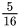to a decimal?Jiaxin

Solution:

Step:1 To convert a fraction into a decimal, following procedure is used.

1. Observe the denominator of the fraction, if the denominator is a number which can be written in the form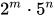, where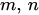are non-negative integers, then the fraction can be converted in a terminating decimal otherwise it is a non-terminating decimal.

2. Divide the numerator by denominator.

3. If the division is non-terminating or the division is too long, then the decimal expansion can be rounded up to 2 to 3 decimal places, which gives the approximate value of fraction.

Step:2 To Similarly, to convert a decimal into fraction, following procedure is used.

1. Observe, after how many places from the right a decimal is placed.

2. Divide the number after removing the decimal from it by a number as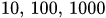etc number of zeros after 1 depends upon the place from the right a decimal is placed.

3. Convert the fraction obtained in step 2 in simplest form.. If the denominator is greater than the numerator then the fraction is proper and if the numerator is greater than denominator then the fraction is improper.

Conversion ofto a decimal:

1.In the fraction, the denominator 16 can be written as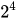.

Therefore, the decimal expansion of above fraction is a terminating decimal.

2. Divide the numerator 5 by 16.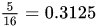3. The exact decimal expansion ofis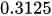.

Answer:isas a decimal.

Similar Problems:

Problem 1: Convert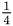as a decimal.

Solution:

Step:1 In the fraction, the denominator 4 can be written as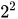.

Step:2 Therefore, the decimal expansion of above fraction is a terminating decimal.

Divide the numerator 1 by 4.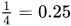Answer:is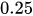as a decimal.

Problem 2: Convert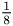as a fraction.

Solution:

Step:1 In the fraction, the denominator 4 can be written as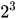.

Step:2 Therefore, the decimal expansion of above fraction is a terminating decimal.

2. Divide the numerator 1 by 8.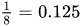Answer:is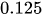as a decimal.

Need homework help now?

tutors online

Related Questions
(t) in °C of the pot of soup at time t (in The initial temperature of a pot of soup is 80°C. The temperature minutes) satisfies the differential equation dT di T-20 10 (a) Solve the differential equation, and find an explicit expression for the function (). By separating variables, integrating and manipulating arbitrary constants, . We are also told that so that and so . ...
Notes
Me

OR

Don't have an account?

Join OneClass

Access over 10 million pages of study
documents for 1.3 million courses.

Join to view

OR

By registering, I agree to the Terms and Privacy Policies
Just a few more details

So we can recommend you notes for your school.